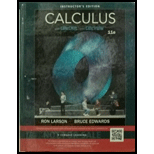Chapter 7, Problem 20RE### Calculus (MindTap Course List)

11th Edition
Ron Larson + 1 other
ISBN: 9781337275347

#### Solutions

Chapter
Section### Calculus (MindTap Course List)

11th Edition
Ron Larson + 1 other
ISBN: 9781337275347
Textbook Problem

# Finding the Volume of a Solid In Exercises 19 and 20, use the disk method to find the volume of the solid generated by revolving the region bounded by the graphs of the equations about the y-axis. y = e − x , y = 0 x = 0 , x = 1

To determine

To calculate: The volume of the solid generated by revolving the region bound by graphs of the equations y=ex,y=0,x=0,x=1 about the x-axisusing disk method.

Explanation

Given:

y=ex isbounded by y=0,x=0,x=1

Formula used:

The disk method states that the volume can be calculated by,

V=πab(f(x))2dx

And the integral:

exdx=ex

Calculation:

Consider the function,

y=ex

Tabular form of the function is:

x012y=ex10.370.14(x,y)(0,1)(1,0

### Still sussing out bartleby?

Check out a sample textbook solution.

See a sample solution

#### The Solution to Your Study Problems

Bartleby provides explanations to thousands of textbook problems written by our experts, many with advanced degrees!

Get Started

## Additional Math Solutions

#### In Exercises 33-38, rewrite the expression using positive exponents only. 35. x1/3x1/2

Applied Calculus for the Managerial, Life, and Social Sciences: A Brief Approach

#### In problems 63-73, factor each expression completely. 69.

Mathematical Applications for the Management, Life, and Social Sciences

#### Show that cosh x1+12x2 for all x.

Single Variable Calculus: Early Transcendentals

#### Finding a Limit In Exercises 11-28, find the limit. limx44x1

Calculus: Early Transcendental Functions (MindTap Course List)